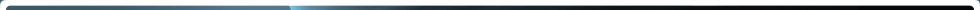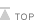Home > NRC Library > Document Collections > NRC Regulations (10 CFR) >  Part Index > § 20.1004 Units of radiation dose.

# § 20.1004 Units of radiation dose.

(a) Definitions. As used in this part, the units of radiation dose are:

Gray (Gy) is the SI unit of absorbed dose. One gray is equal to an absorbed dose of 1 Joule/kilogram (100 rads).

Rad is the special unit of absorbed dose. One rad is equal to an absorbed dose of 100 ergs/gram or 0.01 joule/kilogram (0.01 gray).

Rem is the special unit of any of the quantities expressed as dose equivalent. The dose equivalent in rems is equal to the absorbed dose in rads multiplied by the quality factor (1 rem=0.01 sievert).

Sievert is the SI unit of any of the quantities expressed as dose equivalent. The dose equivalent in sieverts is equal to the absorbed dose in grays multiplied by the quality factor (1 Sv=100 rems).

(b) As used in this part, the quality factors for converting absorbed dose to dose equivalent are shown in table 1004(b).1.

Table 1004(b).1-Quality Factors and Absorbed Dose Equivalencies

Type of radiation Quality factor Absorbed dose equal to a unit dose equivalenta
(Q)
X-, gamma, or beta radiation 1 1
Alpha particles, multiple-charged particles, fission fragments and heavy particles of unknown charge 20 0.05
Neutrons of unknown energy 10 0.1
High-energy protons 10 0.1

a Absorbed dose in rad equal to 1 rem or the absorbed dose in gray equal to 1 sievert.

(c) If it is more convenient to measure the neutron fluence rate than to determine the neutron dose equivalent rate in rems per hour or sieverts per hour, as provided in paragraph (b) of this section, 1 rem (0.01 Sv) of neutron radiation of unknown energies may, for purposes of the regulations in this part, be assumed to result from a total fluence of 25 million neutrons per square centimeter incident upon the body. If sufficient information exists to estimate the approximate energy distribution of the neutrons, the licensee may use the fluence rate per unit dose equivalent or the appropriate Q value from table 1004(b).2 to convert a measured tissue dose in rads to dose equivalent in rems.

Table 1004(b).2.—Mean Quality Factors, Q, and Fluence Per Unit Dose Equivalent for Monoenergetic Neutrons

Neutron energy (MeV) Quality factora (Q) Fluence per unit dose equivalentb
(neutrons cm-2 rem -1)
(thermal)..... 2.5 x 10-8 2 980 x 106
1 x 10-7 2 980 x 106
1 x 10-6 2 810 x 106
1 x 10-5 2 810 x 106
1 x 10-4 2 840 x 106
1 x 10-3 2 980 x 106
1 x 10-2 2.5 1010 x 106
1 x 10-1 7.5 170 x 106
5 x 10-1 11 39 x 106
1 11 27 x 106
2.5 9 29 x 106
5 8 23 x 106
7 7 24 x 106
10 6.5 24 x 106
14 7.5 17 x 106
20 8 16 x 106
40 7 14 x 106
60 5.5 16 x 106
1 x 102 4 20 x 106
2 x 102 3.5 19 x 106
3 x 102 3.5 16 x 106
4 x 102 3.5 14 x 106

a Value of quality factor (Q) at the point where the dose equivalent is maximum in a 30-cm diameter cylinder tissue-equivalent phantom.
b
Monoenergetic neutrons incident normally on a 30-cm diameter cylinder tissue-equivalent phantom.Page Last Reviewed/Updated Friday, August 24, 2018

#### Home